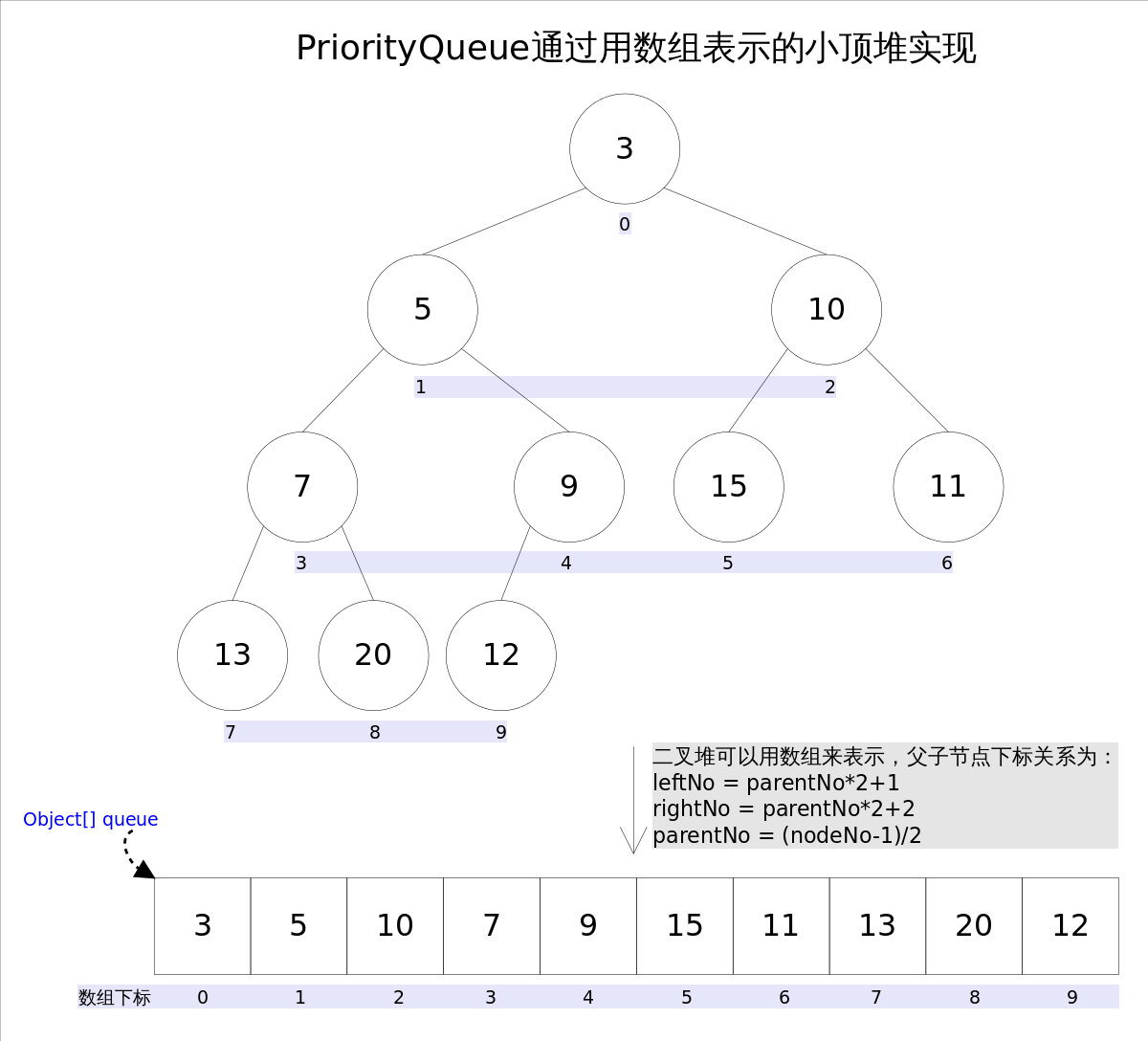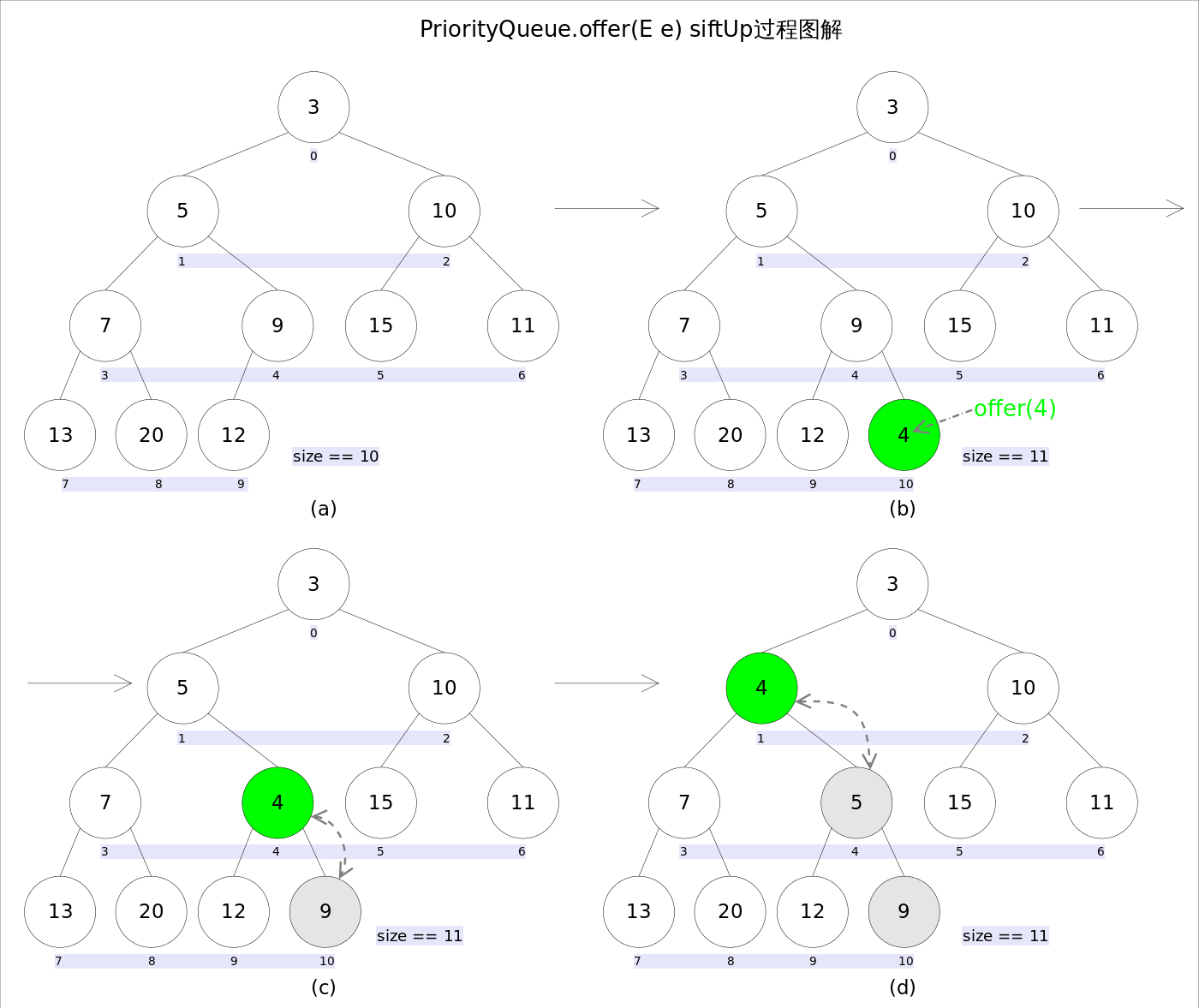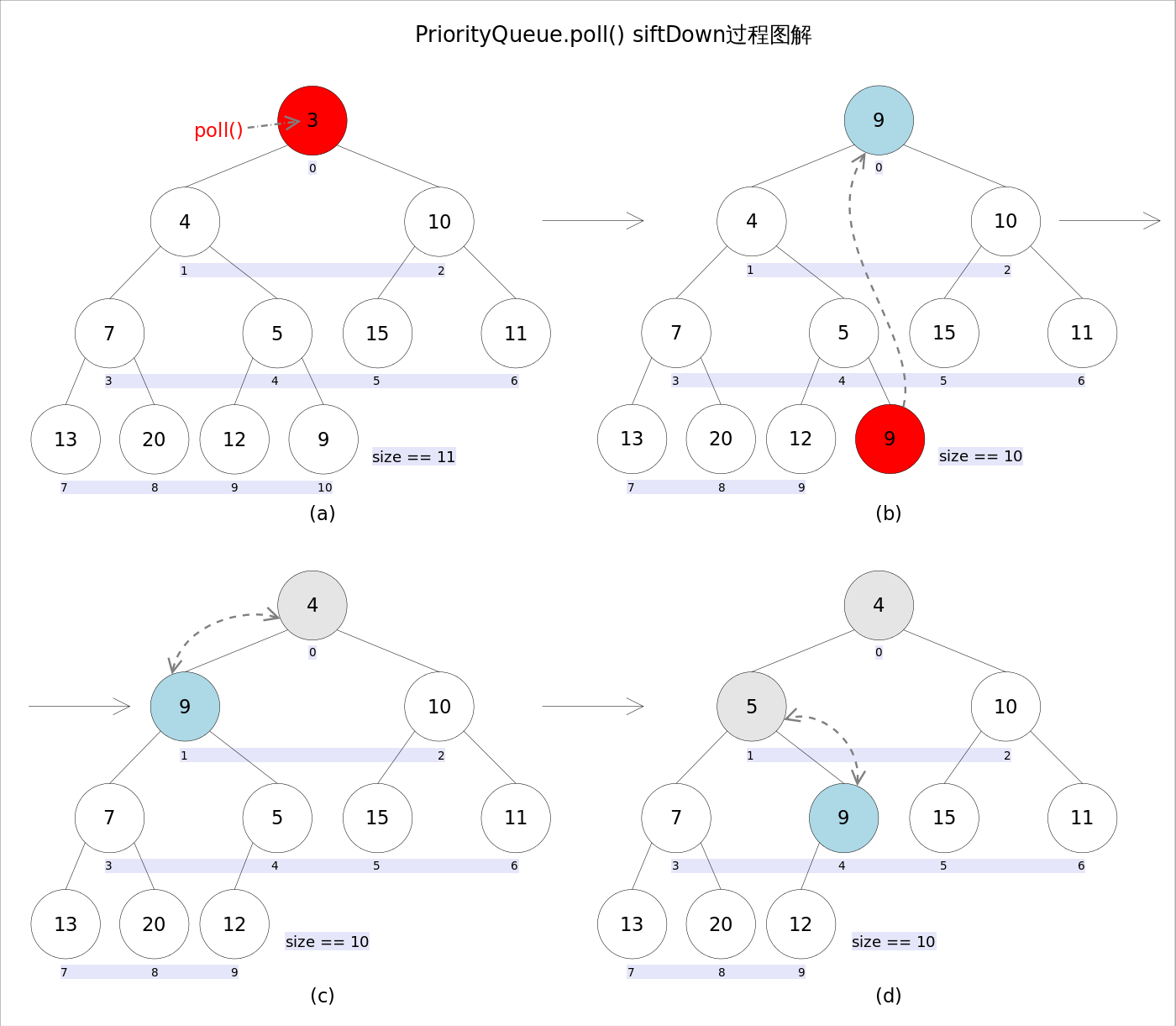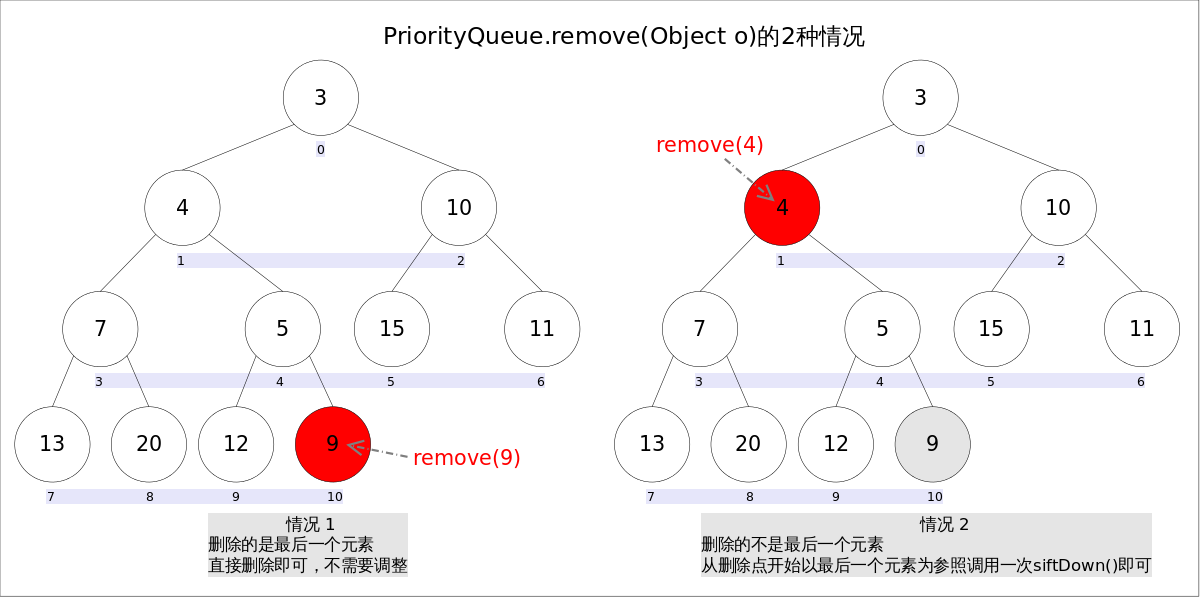# 2.7 PriorityQueue

Wu Jun 2020-01-01 17:43:49
Categories： Tags：

### 1 总体介绍• `leftNo = parentNo*2+1`
• `rightNo = parentNo*2+2`
• `parentNo = (nodeNo-1)/2`

`PriorityQueue``peek()``element`操作是常数时间，`add()`, `offer()`, 无参数的`remove()`以及`poll()`方法的时间复杂度都是log(N)

### 2 方法剖析

`add(E e)``offer(E e)`都是向优先队列中插入元素，只是前者在插入失败时抛出异常，后则则会返回`false```````//offer(E e)
public boolean offer(E e) {
if (e == null)//不允许放入null元素
throw new NullPointerException();
modCount++;
int i = size;
if (i >= queue.length)
grow(i + 1);//自动扩容
size = i + 1;
if (i == 0)//队列原来为空，这是插入的第一个元素
queue = e;
else
siftUp(i, e);//调整
return true;
}
``````

##### 1）调整

`siftUp(int k, E x)`方法，用于插入元素`x`并维持堆的特性。调整的过程为：从`k`指定的位置开始，将`x`逐层与当前点的`parent`进行比较并交换，直到满足`x >= queue[parent]`为止。

``````//siftUp()
private void siftUp(int k, E x) {
while (k > 0) {
int parent = (k - 1) >>> 1;//parentNo = (nodeNo-1)/2
Object e = queue[parent];
if (comparator.compare(x, (E) e) >= 0)//调用比较器的比较方法
break;
queue[k] = e;
k = parent;
}
queue[k] = x;
}
``````

#### 2.2 element() 和 peek()

`element()``peek()`都是获取但不删除队首元素，区别是当方法失败时前者抛出异常，后者返回`null`直接返回数组`0`下标处的那个元素即可

#### 2.3 remove() 和 poll()

`remove()``poll()`都是获取并删除队首元素，区别是当方法失败时前者抛出异常，后者返回`null`。由于删除操作会改变队列的结构，为维护小顶堆的性质，需要进行必要的调整。``````public E poll() {
if (size == 0)
return null;
int s = --size;
modCount++;
E result = (E) queue;//0下标处的那个元素就是最小的那个
E x = (E) queue[s];
queue[s] = null;
if (s != 0)
siftDown(0, x);//调整
return result;
}
``````

##### 1）调整

`siftDown(int k, E x)`方法，作用是从`k`指定的位置开始，将`x`逐层向下与当前点的左右孩子中较小的那个交换，直到`x`小于或等于左右孩子中的任何一个为止。

``````//siftDown()
private void siftDown(int k, E x) {
int half = size >>> 1;
while (k < half) {
//首先找到左右孩子中较小的那个，记录到c里，并用child记录其下标
int child = (k << 1) + 1;//leftNo = parentNo*2+1
Object c = queue[child];
int right = child + 1;
if (right < size &&
comparator.compare((E) c, (E) queue[right]) > 0)
c = queue[child = right];
if (comparator.compare(x, (E) c) <= 0)
break;
queue[k] = c;//然后用c取代原来的值
k = child;
}
queue[k] = x;
}
``````

#### 2.4 remove(Object o)

`remove(Object o)`方法用于删除队列中跟`o`相等的某一个元素（如果有多个相等，只删除一个），该方法不是Queue接口内的方法，而是Collection接口的方法。

`remove(Object o)`可以分为2种情况：

1. 删除的是最后一个元素。直接删除即可，不需要调整。
2. 删除的不是最后一个元素，从删除点开始以最后一个元素为参照调用一次`siftDown()`即可。``````//remove(Object o)
public boolean remove(Object o) {
//通过遍历数组的方式找到第一个满足o.equals(queue[i])元素的下标
int i = indexOf(o);
if (i == -1)
return false;
int s = --size;
if (s == i) //情况1
queue[i] = null;
else {
E moved = (E) queue[s];
queue[s] = null;
siftDown(i, moved);//情况2
......
}
return true;
}
``````